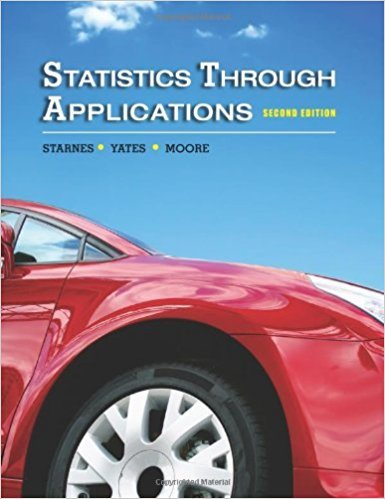×
Get Full Access to Statistics Through Applications - 2 Edition - Chapter 3 - Problem 3.26
Get Full Access to Statistics Through Applications - 2 Edition - Chapter 3 - Problem 3.26

×

# Eggs A truck is loaded with cartons of eggs that weigh anISBN: 9781429219747 133

## Solution for problem 3.26 Chapter 3

Statistics Through Applications | 2nd Edition

• Textbook Solutions
• 2901 Step-by-step solutions solved by professors and subject experts
• Get 24/7 help from StudySoup virtual teaching assistantsStatistics Through Applications | 2nd Edition

4 5 1 424 Reviews
28
3
Problem 3.26

Eggs A truck is loaded with cartons of eggs that weigh an average of 2 pounds each with a standard deviation of 0.1 pound. A histogram of these weights looks very much like a Normal distribution. Use the 689599.7 rule to answer the following questions. (a) What percent of the cartons weigh less than 2.1 pounds? Show your work. (b) What percent of the cartons weigh less than 1.8 pounds? Show your work. (c) What percent of the cartons weigh more than 1.9 pounds? Show your work.

Step-by-Step Solution:
Step 1 of 3

Math 112 TUESDAY Syllabus Fall 2017 COURSE TITLE: Precalculus Algebra INSTRUCTOR: SECTION: _________ PREREQUISITE: Grade of “C-“or better in Math 100 or a minimum of 310 on the math placement test. CREDIT: 3 semester hours COURSE DESCRIPTION: A higher-level course emphasizing functions including polynomial functions, rational functions, and the exponential and logarithmic functions. Graphs of these functions are stressed. The course also includes work on equations, inequalities, systems of equations, and the complex and rational roots of polynomials. Applications are stressed. This is a course in Precalculus mathematics. The course  Is computer-assisted, which means students will use the computer (along with the instructor) to complete the cou

Step 2 of 3

Step 3 of 3

##### ISBN: 9781429219747

Unlock Textbook Solution